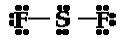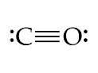# Solutions 17

$$\newcommand{\vecs}{\overset { \rightharpoonup} {\mathbf{#1}} }$$ $$\newcommand{\vecd}{\overset{-\!-\!\rightharpoonup}{\vphantom{a}\smash {#1}}}$$$$\newcommand{\id}{\mathrm{id}}$$ $$\newcommand{\Span}{\mathrm{span}}$$ $$\newcommand{\kernel}{\mathrm{null}\,}$$ $$\newcommand{\range}{\mathrm{range}\,}$$ $$\newcommand{\RealPart}{\mathrm{Re}}$$ $$\newcommand{\ImaginaryPart}{\mathrm{Im}}$$ $$\newcommand{\Argument}{\mathrm{Arg}}$$ $$\newcommand{\norm}{\| #1 \|}$$ $$\newcommand{\inner}{\langle #1, #2 \rangle}$$ $$\newcommand{\Span}{\mathrm{span}}$$ $$\newcommand{\id}{\mathrm{id}}$$ $$\newcommand{\Span}{\mathrm{span}}$$ $$\newcommand{\kernel}{\mathrm{null}\,}$$ $$\newcommand{\range}{\mathrm{range}\,}$$ $$\newcommand{\RealPart}{\mathrm{Re}}$$ $$\newcommand{\ImaginaryPart}{\mathrm{Im}}$$ $$\newcommand{\Argument}{\mathrm{Arg}}$$ $$\newcommand{\norm}{\| #1 \|}$$ $$\newcommand{\inner}{\langle #1, #2 \rangle}$$ $$\newcommand{\Span}{\mathrm{span}}$$

## S17.1

(a) eight electrons:;

(b) eight electrons:;

(c) no electrons

Be2+;

(d) eight electrons:;

(e) no electrons

Ga3+;

(f) no electrons

Li+;

(g) eight electrons:## S17.2

Write Lewis structures for the following:

a. H2b. HBrc. PCl3d. SF2e. H2CCH2f. HNNHg. H2CNHh. NOi. N2j. COk. CN## S17.3

aWhen H and Cl are separate (the x axis) the energy is at a particular value. As they approach, it decreases to a minimum at 127 pm (the bond distance), and then it increases sharply as you get closer.

You can also find the potential energy function of H-Cl and take the derivative with respect to internuclear distance and find minimum.

1. $$H–Cl$$: $$energy of one bond=431 kJ/mol /N_A=431 \times 10^3 J/mol x \dfrac{1 mol}{6.02 \times 10^{23} bonds}=7.16 \times 10^{-19} J/bond$$

## S17.4

The single bond present in each molecule results from overlap of the relevant orbitals: F 2p orbitals in F2, the H 1s and F 2p orbitals in HF, and the Cl 3p orbital and Br 4p orbital in ClBr.

## S17.5

$$\ce{H–C≡N}$$ has two σ (H–C and C–N) and two π (making the CN triple bond).## S17.6

An ionic bond wave function takes into account the probability that the electrons of a multiatomic molecule might exist on the same atom; the covalent bond wave function assumes they exist on separate atoms.

Solutions 17 is shared under a not declared license and was authored, remixed, and/or curated by LibreTexts.